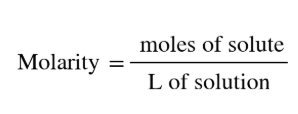# Problem: A strontium hydroxide solution is prepared by dissolving 10.45 g of Sr(OH)2 in water to make 53.00 mL of solution.What is the molarity of this solution?

###### FREE Expert Solution
• Molarity can be calculated as:• Setup a dimensional analysis where we start with the mass of Sr(OH)2 converting it to moles using its MM. Then divide it by the volume in L
87% (66 ratings)###### Problem Details

A strontium hydroxide solution is prepared by dissolving 10.45 g of Sr(OH)2 in water to make 53.00 mL of solution.What is the molarity of this solution?